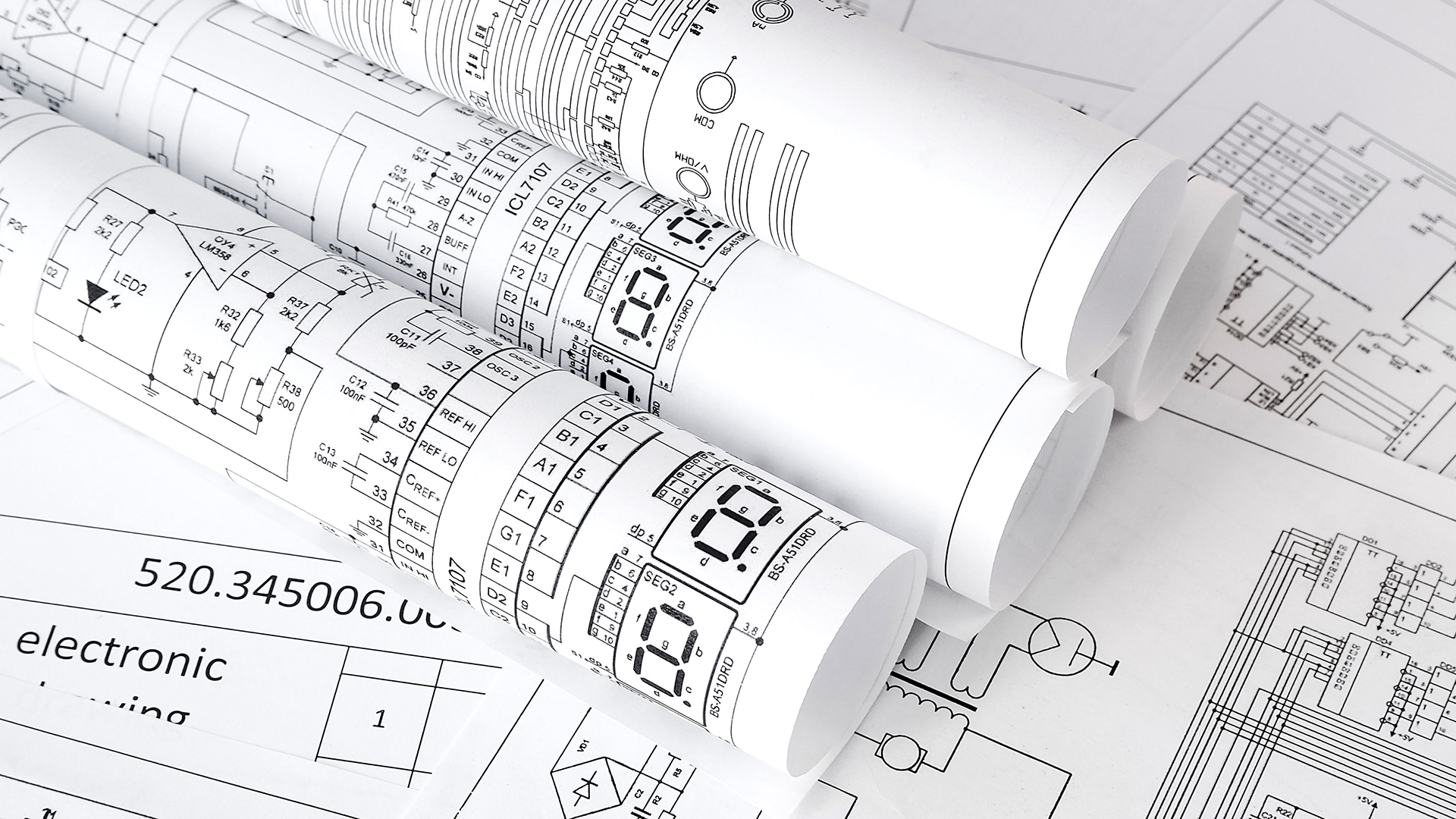# 611-04 - Logic Diagrams## Learning objectives

• Explain the purpose of a logic diagram
• Describe the title block, revision block, reference block, legend block, and grid coordinates
• Identify the common symbols associated with logic diagrams
• Describe the principles of logic gates and identify common logic gate types
• Define the following terms: logic gates, Boolean logic, ladder logic, truth tables

## Course overview

When you complete this lesson, you will be able to state the important features of a logic diagram and identify the most common types of logic gates and how each is used. You will also be able to identify the common symbols and omponents on a logic diagram and explain their relationship to each other.
• 40 minutes
• Format: Online Interactive
• English